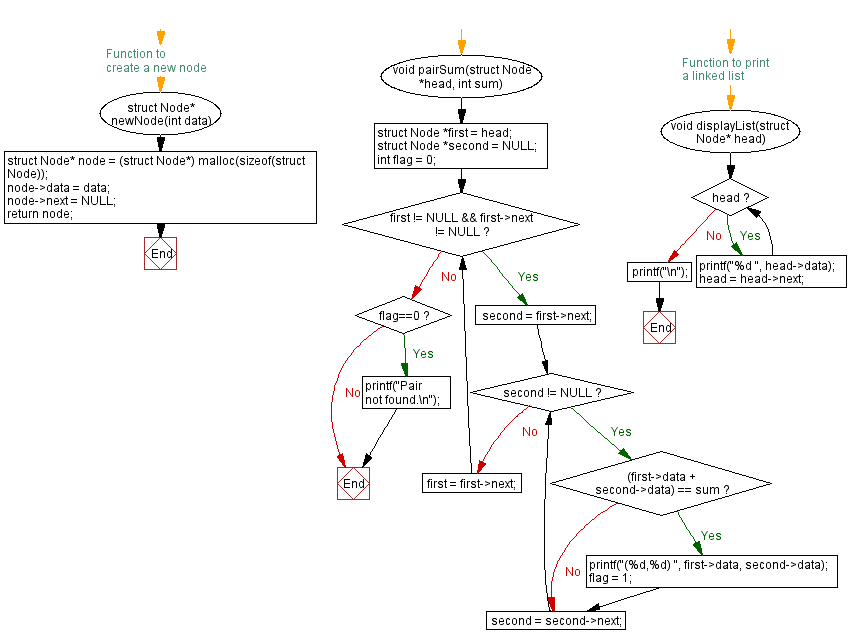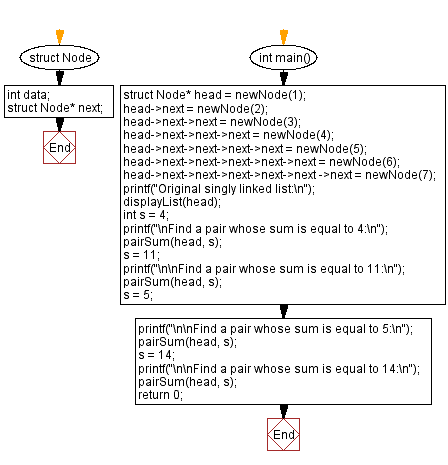﻿ C Program: Pair in a linked list whose sum is equal to a given value

# C Exercises: Pair in a linked list whose sum is equal to a given value

## C Singly Linked List : Exercise-38 with Solution

Write a C program to find a pair in a singly linked list whose sum is equal to a given value.

Sample Solution:

C Code:

``````#include<stdio.h>
#include <stdlib.h>

struct Node {
int data;
struct Node* next;
};

// Function to create a new node
struct Node* newNode(int data) {
struct Node* node = (struct Node*) malloc(sizeof(struct Node));
node->data = data;
node->next = NULL;
return node;
}
void pairSum(struct Node *head, int sum) {
struct Node *second = NULL;
int flag = 0;
while (first != NULL && first->next != NULL) {
second = first->next;

while (second != NULL) {
if ((first->data + second->data) == sum) {
printf("(%d,%d) ", first->data, second->data);
flag = 1;
}
second = second->next;
}

first = first->next;
}
if(flag==0)
}

// Function to print a linked list
}
printf("\n");
}

int main() {
int s = 4;
printf("\nFind a pair whose sum is equal to 4:\n");
s = 11;
printf("\n\nFind a pair whose sum is equal to 11:\n");
s = 5;
printf("\n\nFind a pair whose sum is equal to 5:\n");
s = 14;
printf("\n\nFind a pair whose sum is equal to 14:\n");

return 0;
}
```
```

Sample Output:

```Original singly linked list:
1 2 3 4 5 6 7

Find a pair whose sum is equal to 4:
(1,3)

Find a pair whose sum is equal to 11:
(4,7) (5,6)

Find a pair whose sum is equal to 5:
(1,4) (2,3)

Find a pair whose sum is equal to 14:
```

Flowchart :C Programming Code Editor:

What is the difficulty level of this exercise?

﻿